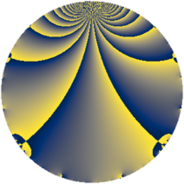# Properties

 Label 1014.2.aLevel $1014$ Weight $2$ Character orbit 1014.a Rep. character $\chi_{1014}(1,\cdot)$ Character field $\Q$ Dimension $27$ Newform subspaces $15$ Sturm bound $364$ Trace bound $5$

# Related objects

## Defining parameters

 Level: $$N$$ $$=$$ $$1014 = 2 \cdot 3 \cdot 13^{2}$$ Weight: $$k$$ $$=$$ $$2$$ Character orbit: $$[\chi]$$ $$=$$ 1014.a (trivial) Character field: $$\Q$$ Newform subspaces: $$15$$ Sturm bound: $$364$$ Trace bound: $$5$$ Distinguishing $$T_p$$: $$5$$, $$7$$

## Dimensions

The following table gives the dimensions of various subspaces of $$M_{2}(\Gamma_0(1014))$$.

Total New Old
Modular forms 210 27 183
Cusp forms 155 27 128
Eisenstein series 55 0 55

The following table gives the dimensions of the cuspidal new subspaces with specified eigenvalues for the Atkin-Lehner operators and the Fricke involution.

$$2$$$$3$$$$13$$FrickeDim.
$$+$$$$+$$$$+$$$$+$$$$1$$
$$+$$$$+$$$$-$$$$-$$$$5$$
$$+$$$$-$$$$+$$$$-$$$$4$$
$$+$$$$-$$$$-$$$$+$$$$3$$
$$-$$$$+$$$$+$$$$-$$$$5$$
$$-$$$$+$$$$-$$$$+$$$$2$$
$$-$$$$-$$$$+$$$$+$$$$1$$
$$-$$$$-$$$$-$$$$-$$$$6$$
Plus space$$+$$$$7$$
Minus space$$-$$$$20$$

## Trace form

 $$27 q + q^{2} + q^{3} + 27 q^{4} - 2 q^{5} - q^{6} - 4 q^{7} + q^{8} + 27 q^{9} + O(q^{10})$$ $$27 q + q^{2} + q^{3} + 27 q^{4} - 2 q^{5} - q^{6} - 4 q^{7} + q^{8} + 27 q^{9} + 2 q^{10} + 4 q^{11} + q^{12} + 8 q^{14} + 2 q^{15} + 27 q^{16} + 2 q^{17} + q^{18} + 8 q^{19} - 2 q^{20} + 4 q^{21} + 8 q^{23} - q^{24} + 37 q^{25} + q^{27} - 4 q^{28} + 6 q^{29} - 2 q^{30} + 4 q^{31} + q^{32} - 4 q^{33} + 2 q^{34} + 27 q^{36} + 2 q^{37} - 4 q^{38} + 2 q^{40} + 10 q^{41} - 4 q^{42} + 4 q^{43} + 4 q^{44} - 2 q^{45} - 8 q^{47} + q^{48} + 31 q^{49} - q^{50} + 10 q^{51} + 22 q^{53} - q^{54} + 16 q^{55} + 8 q^{56} - 8 q^{57} + 6 q^{58} - 4 q^{59} + 2 q^{60} + 14 q^{61} - 4 q^{63} + 27 q^{64} + 4 q^{66} + 16 q^{67} + 2 q^{68} + 8 q^{69} + 8 q^{70} + 8 q^{71} + q^{72} - 2 q^{73} - 2 q^{74} - q^{75} + 8 q^{76} + 24 q^{77} + 12 q^{79} - 2 q^{80} + 27 q^{81} - 6 q^{82} - 12 q^{83} + 4 q^{84} - 4 q^{85} + 4 q^{86} + 10 q^{87} - 14 q^{89} + 2 q^{90} + 8 q^{92} - 4 q^{93} + 16 q^{94} + 32 q^{95} - q^{96} - 10 q^{97} + 9 q^{98} + 4 q^{99} + O(q^{100})$$

## Decomposition of $$S_{2}^{\mathrm{new}}(\Gamma_0(1014))$$ into newform subspaces

Label Dim $A$ Field CM Traces A-L signs $q$-expansion
$a_{2}$ $a_{3}$ $a_{5}$ $a_{7}$ 2 3 13
1014.2.a.a $1$ $8.097$ $$\Q$$ None $$-1$$ $$-1$$ $$-1$$ $$-2$$ $+$ $+$ $+$ $$q-q^{2}-q^{3}+q^{4}-q^{5}+q^{6}-2q^{7}+\cdots$$
1014.2.a.b $1$ $8.097$ $$\Q$$ None $$-1$$ $$1$$ $$-2$$ $$-2$$ $+$ $-$ $-$ $$q-q^{2}+q^{3}+q^{4}-2q^{5}-q^{6}-2q^{7}+\cdots$$
1014.2.a.c $1$ $8.097$ $$\Q$$ None $$-1$$ $$1$$ $$3$$ $$2$$ $+$ $-$ $+$ $$q-q^{2}+q^{3}+q^{4}+3q^{5}-q^{6}+2q^{7}+\cdots$$
1014.2.a.d $1$ $8.097$ $$\Q$$ None $$1$$ $$-1$$ $$-2$$ $$-4$$ $-$ $+$ $+$ $$q+q^{2}-q^{3}+q^{4}-2q^{5}-q^{6}-4q^{7}+\cdots$$
1014.2.a.e $1$ $8.097$ $$\Q$$ None $$1$$ $$-1$$ $$1$$ $$2$$ $-$ $+$ $+$ $$q+q^{2}-q^{3}+q^{4}+q^{5}-q^{6}+2q^{7}+\cdots$$
1014.2.a.f $1$ $8.097$ $$\Q$$ None $$1$$ $$1$$ $$-3$$ $$-2$$ $-$ $-$ $+$ $$q+q^{2}+q^{3}+q^{4}-3q^{5}+q^{6}-2q^{7}+\cdots$$
1014.2.a.g $1$ $8.097$ $$\Q$$ None $$1$$ $$1$$ $$2$$ $$2$$ $-$ $-$ $-$ $$q+q^{2}+q^{3}+q^{4}+2q^{5}+q^{6}+2q^{7}+\cdots$$
1014.2.a.h $2$ $8.097$ $$\Q(\sqrt{3})$$ None $$-2$$ $$-2$$ $$0$$ $$6$$ $+$ $+$ $-$ $$q-q^{2}-q^{3}+q^{4}+\beta q^{5}+q^{6}+(3-\beta )q^{7}+\cdots$$
1014.2.a.i $2$ $8.097$ $$\Q(\sqrt{3})$$ None $$-2$$ $$2$$ $$-4$$ $$2$$ $+$ $-$ $-$ $$q-q^{2}+q^{3}+q^{4}+(-2+\beta )q^{5}-q^{6}+\cdots$$
1014.2.a.j $2$ $8.097$ $$\Q(\sqrt{3})$$ None $$2$$ $$-2$$ $$0$$ $$-6$$ $-$ $+$ $-$ $$q+q^{2}-q^{3}+q^{4}+\beta q^{5}-q^{6}+(-3+\cdots)q^{7}+\cdots$$
1014.2.a.k $2$ $8.097$ $$\Q(\sqrt{3})$$ None $$2$$ $$2$$ $$4$$ $$-2$$ $-$ $-$ $-$ $$q+q^{2}+q^{3}+q^{4}+(2+\beta )q^{5}+q^{6}+\cdots$$
1014.2.a.l $3$ $8.097$ $$\Q(\zeta_{14})^+$$ None $$-3$$ $$-3$$ $$-1$$ $$-9$$ $+$ $+$ $-$ $$q-q^{2}-q^{3}+q^{4}+(1-3\beta _{1}+\beta _{2})q^{5}+\cdots$$
1014.2.a.m $3$ $8.097$ $$\Q(\zeta_{14})^+$$ None $$-3$$ $$3$$ $$3$$ $$-3$$ $+$ $-$ $+$ $$q-q^{2}+q^{3}+q^{4}+(1+\beta _{1}+\beta _{2})q^{5}+\cdots$$
1014.2.a.n $3$ $8.097$ $$\Q(\zeta_{14})^+$$ None $$3$$ $$-3$$ $$1$$ $$9$$ $-$ $+$ $+$ $$q+q^{2}-q^{3}+q^{4}+(-\beta _{1}-2\beta _{2})q^{5}+\cdots$$
1014.2.a.o $3$ $8.097$ $$\Q(\zeta_{14})^+$$ None $$3$$ $$3$$ $$-3$$ $$3$$ $-$ $-$ $-$ $$q+q^{2}+q^{3}+q^{4}+(-1-\beta _{1}-\beta _{2})q^{5}+\cdots$$

## Decomposition of $$S_{2}^{\mathrm{old}}(\Gamma_0(1014))$$ into lower level spaces

$$S_{2}^{\mathrm{old}}(\Gamma_0(1014)) \cong$$ $$S_{2}^{\mathrm{new}}(\Gamma_0(26))$$$$^{\oplus 4}$$$$\oplus$$$$S_{2}^{\mathrm{new}}(\Gamma_0(39))$$$$^{\oplus 4}$$$$\oplus$$$$S_{2}^{\mathrm{new}}(\Gamma_0(78))$$$$^{\oplus 2}$$$$\oplus$$$$S_{2}^{\mathrm{new}}(\Gamma_0(169))$$$$^{\oplus 4}$$$$\oplus$$$$S_{2}^{\mathrm{new}}(\Gamma_0(338))$$$$^{\oplus 2}$$$$\oplus$$$$S_{2}^{\mathrm{new}}(\Gamma_0(507))$$$$^{\oplus 2}$$